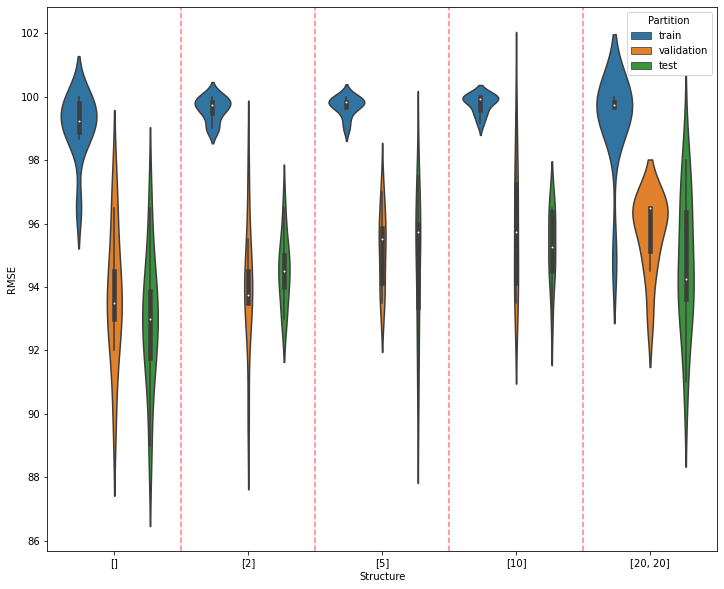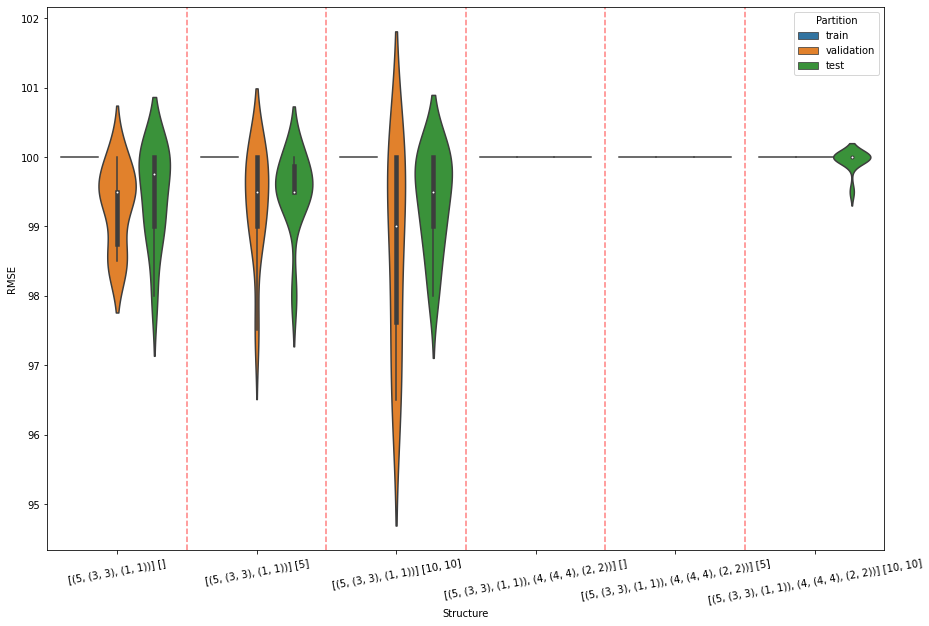Neural Networks in Pytorch

In this assignment, you will

1. complete the implementations of neural network classes,
2. define the new function multiple_runs_classification,
3. define the new function multiple_runs_convolutional,
4. copy and paste percent_correct and confusion_matrx from previous notes,
5. present and discuss regression results on automobile MPG data,
6. present and discuss classification results on diabetes data,
7. present and discuss classification results on small version of MNIST data.

# Neural Network Classes¶

In :
import numpy as np
import matplotlib.pyplot as plt
import torch


• NeuralNetworkTorch: copied and pasted from Lecture Notes 16.1
• NeuralNetworkClassifierTorch: copied and pasted from Lecture Notes 16.1
• NeuralNetworkClassifierConvolutionalTorch: copied and pasted from Lecture Notes 16.1 and completed.

The train functions in this classes have two additional arguments, Xvalnad Tval:

def train(self, Xtrain, Ttrain, n_epochs, learning_rate=0.01, method='adam', verbose=True,
Xval=None, Tval=None):


where Xtrain and Ttrain are the usual two arguments used to train the network, and Xval and Tval are the validation data matrices. Xval and Tval are only used to calculate the performance (MSE or NLL) once per epoch. If the performance is better than the best so far, update an array that is storing the best weight values so far, and also update the best performance so far. When training is complete, copy the stored best-so-far weights back into the network. If Xval is None, then this step is ignored and the weights in the neural network at the end of training are not modified.

Include in this notebook the following functions we have defined before

• percent_correct
• partition
• multiple_runs_regression

and define two new functions

• multiple_runs_classification
• multiple_runs_convolutional

based on multiple_runs_regression.

Here is another function that you must use to analyze the performance for your two classification problems.

In :
def confusion_matrix(Y_classes, T):
class_names = np.unique(T)
table = []
for true_class in class_names:
row = []
for Y_class in class_names:
row.append(100 * np.mean(Y_classes[T == true_class] == Y_class))
table.append(row)
conf_matrix = pd.DataFrame(table, index=class_names, columns=class_names)
print(f'Percent Correct is {percent_correct(Y_classes, T)}')
return conf_matrix


Here are examples of running multiple_runs_classification and multiple_runs_convolutional.

In :
def makeImages(nEach):
images = np.zeros((nEach * 2, 1, 20, 20))  # nSamples, nChannels, rows, columns
radii = 3 + np.random.randint(10 - 5, size=(nEach * 2, 1))
centers = np.zeros((nEach * 2, 2))
for i in range(nEach * 2):
centers[i, :] = r + 1 + np.random.randint(18 - 2 * r, size=(1, 2))
x = int(centers[i, 0])
y = int(centers[i, 1])
if i < nEach:
# squares
images[i, 0, x - r:x + r, y + r] = 1.0
images[i, 0, x - r:x + r, y - r] = 1.0
images[i, 0, x - r, y - r:y + r] = 1.0
images[i, 0, x + r, y - r:y + r + 1] = 1.0
else:
# diamonds
images[i, 0, range(x - r, x), range(y, y + r)] = 1.0
images[i, 0, range(x - r, x), range(y, y - r, -1)] = 1.0
images[i, 0, range(x, x + r + 1), range(y + r, y - 1, -1)] = 1.0
images[i, 0, range(x, x + r), range(y - r, y)] = 1.0
# images += np.random.randn(*images.shape) * 0.5
T = np.zeros((nEach * 2, 1))
T[nEach:] = 1
return images, T

nEach = 500
X, T = makeImages(nEach)
Xflat = X.reshape(X.shape, -1)
Xflat.shape, T.shape

Out:
((1000, 400), (1000, 1))
In :
fractions = (0.6, 0.2, 0.2)
n_hiddens_list = [[], , , , [20, 20]]  # Notice the first one... []
n_epochs = 500
learning_rate = 0.01

n_partitions = 10

results = []
for nh in n_hiddens_list:
results.extend(multiple_runs_classification(n_partitions, Xflat, T, fractions, nh, n_epochs, learning_rate))

resultsdf = pd.DataFrame(results, columns=('Structure', 'Partition', 'RMSE'))

Structure []: Repetition 1 2 3 4 5 6 7 8 9 10
Structure : Repetition 1 2 3 4 5 6 7 8 9 10
Structure : Repetition 1 2 3 4 5 6 7 8 9 10
Structure : Repetition 1 2 3 4 5 6 7 8 9 10
Structure [20, 20]: Repetition 1 2 3 4 5 6 7 8 9 10

In :
import seaborn as sns

plt.figure(figsize=(12, 10))
sns.violinplot(x='Structure', y='RMSE', hue='Partition', data=resultsdf)
for x in range(len(n_hiddens_list) - 1):
plt.axvline(x + 0.5, color='r', linestyle='--', alpha=0.5)Based on results in violin plots, pick best network structure (according to performance in validation data) and train a network using that structure. Then apply the trained network using nnet.use on the training, validation and testing partitions. Report the performance of each, and, for classification problems, show the confusion matrices. See examples below.

In :
nnet = NeuralNetworkClassifierTorch(Xflat.shape, , len(np.unique(T)))
Xtrain, Ttrain, Xval, Tval, Xtest, Ttest = partition(Xflat, T, (0.6, 0.2, 0.2),
shuffle=True, classification=True)
nnet.train(Xtrain, Ttrain, n_epochs, learning_rate, method='adam', Xval=Xval, Tval=Tval, verbose=True)

Epoch 50 LL train 0.9950 val 0.8856
Epoch 100 LL train 0.9983 val 0.8740
Epoch 150 LL train 0.9990 val 0.8687
Epoch 200 LL train 0.9993 val 0.8653
Epoch 250 LL train 0.9994 val 0.8621
Epoch 300 LL train 0.9995 val 0.8592
Epoch 350 LL train 0.9996 val 0.8565
Epoch 400 LL train 0.9997 val 0.8540
Epoch 450 LL train 0.9997 val 0.8517
Epoch 500 LL train 0.9998 val 0.8496

Out:
NeuralNetworkClassifierTorch(400, , 2, device=cpu)
In :
C_train, P_train = nnet.use(Xtrain)
confusion_matrix(C_train, Ttrain)

Percent Correct is 100.0

Out:
0.0 1.0
0.0 100.0 0.0
1.0 0.0 100.0
In :
C_val, P_val = nnet.use(Xval)
confusion_matrix(C_val, Tval)

Percent Correct is 97.0

Out:
0.0 1.0
0.0 96.0 4.0
1.0 2.0 98.0
In :
C_test, P_test = nnet.use(Xtest)
confusion_matrix(C_test, Ttest)

Percent Correct is 95.5

Out:
0.0 1.0
0.0 92.0 8.0
1.0 1.0 99.0
In :
fractions = (0.6, 0.2, 0.2)
n_conv_list = [ [(5, (3, 3), (1, 1))],
[(5, (3, 3), (1, 1)), (4, (4, 4), (2, 2))]
]
n_fc_list = [[], , [10, 10]]  # Notice the first one... []
n_epochs = 200
learning_rate = 0.01

n_partitions = 10

results = []
for nconv in n_conv_list:
for nfc in n_fc_list:
results.extend(multiple_runs_convolutional(n_partitions, X, T, fractions, nconv, nfc, n_epochs, learning_rate))

resultsdf = pd.DataFrame(results, columns=('Structure', 'Partition', 'RMSE'))

Conv [(5, (3, 3), (1, 1))] FC []: Repetition 1 2 3 4 5 6 7 8 9 10
Conv [(5, (3, 3), (1, 1))] FC : Repetition 1 2 3 4 5 6 7 8 9 10
Conv [(5, (3, 3), (1, 1))] FC [10, 10]: Repetition 1 2 3 4 5 6 7 8 9 10
Conv [(5, (3, 3), (1, 1)), (4, (4, 4), (2, 2))] FC []: Repetition 1 2 3 4 5 6 7 8 9 10
Conv [(5, (3, 3), (1, 1)), (4, (4, 4), (2, 2))] FC : Repetition 1 2 3 4 5 6 7 8 9 10
Conv [(5, (3, 3), (1, 1)), (4, (4, 4), (2, 2))] FC [10, 10]: Repetition 1 2 3 4 5 6 7 8 9 10

In :
import seaborn as sns

plt.figure(figsize=(15, 10))
sns.violinplot(x='Structure', y='RMSE', hue='Partition', data=resultsdf)
plt.xticks(rotation=10)

for x in range(len(n_conv_list) * len(n_fc_list) - 1):
plt.axvline(x + 0.5, color='r', linestyle='--', alpha=0.5)# Application to Three Data Sets¶

In the following experiments, you may try a variety of learning_rates, numbers of epochs, and network structures, but in the violin plot use one value for learning_rate, one value for number of epochs, and six values of network structure.

Apply multiple_runs_regression for various network structures to the automobile data introduced in Lecture Notes 17. Load it as follows.

In :
import pandas as pd
import os

if os.path.isfile('automobile.csv'):
else:
usecols=range(8))
automobile.columns = ('mpg', 'cylinders', 'displacement', 'horsepower', 'weight',
'acceleration', 'year', 'origin')

print(f'Number rows in original data file {len(automobile)}.')
automobile = automobile.dropna(axis=0)
print(f'Number rows after dropping rows with missing values {len(automobile)}.')
automobile.to_csv('automobile.csv', index=False)  # so row numbers are not written to file

T = automobile['mpg'].values.reshape(-1, 1)
X = automobile.iloc[:, 1:].values
X.shape, T.shape

Reading data from 'automobile.csv'.

Out:
((392, 7), (392, 1))

Apply multiple_runs_classification for various network structures to the following data set from a study in diabetes diagnosis.

In :
if os.path.isfile('diabetes.csv'):
else:
# usecols=range(1,14))
if False:
print(f'Number rows in original data file {len(diabetes)}.')
diabetes = diabetes.dropna(axis=0)
print(f'Number rows after dropping rows with missing values {len(diabetes)}.')
converter = dict(Yes=1, No=0, Female=1, Male=0, Positive=1, Negative=0)
diabetes = diabetes.applymap(lambda x: converter.get(x, x))
diabetes.to_csv('diabetes.csv', index=False)  # so row numbers are not written to file

X = diabetes.iloc[:, :-1].values
T = diabetes.iloc[:, -1:].values

X.shape, T.shape

Downloading diabetes_data_upload.csv from UCI ML Repository.

Out:
((520, 16), (520, 1))

Apply multiple_runs_convolutional for various network structures to the following small subset of the MNIST data that contains 500 samples of each digit.

In :
if os.path.isfile('small_mnist.npz'):
else:
import shlex
import subprocess
cmd = 'curl "https://www.cs.colostate.edu/~anderson/cs545/notebooks/small_mnist.npz" -o "small_mnist.npz"'
subprocess.call(shlex.split(cmd))

X = small_mnist['X']
X = X.reshape(-1, 1, 28, 28)
T = small_mnist['T']

X.shape, T.shape

Downloading small_mnist.npz from CS545 site.

Out:
((1000, 1, 28, 28), (1000, 1))

For each of the three applications, make violin plots. For classification problems, also show the confusion matrices. Describe the results you see with at least 10 sentences for each application, for a total of at least 30 sentences.

Download A5grader.tar, extract A5grader.py before running the following cell.

In :
%run -i A5grader.py

======================= Code Execution =======================

Extracting python code from notebook named 'Anderson-A5.ipynb' and storing in notebookcode.py
Removing all statements that are not function or class defs or import statements.
CRITICAL ERROR: Function named 'rmse' is not defined
Check the spelling and capitalization of the function name.
CRITICAL ERROR: Function named 'multiple_runs_classifiation' is not defined
Check the spelling and capitalization of the function name.
CRITICAL ERROR: Function named 'train_for_best_validation' is not defined
Check the spelling and capitalization of the function name.

## Testing constructor ####################################################################

nnet = NeuralNetworkClassifierConvolutionalTorch([1, 10, 10],
[(2, (3, 3), (1, 1)), (3, (5, 5), (2, 2))],
[30, 20], 2)

# Is isinstance(nnet, NeuralNetworkClassifierTorch)   True?

--- 10/10 points. NeuralNetworkClassifierConvolutionalTorch is correctly of type NeuralNetworkClassifierTorch

--- 10/10 points. nnet correctly has 5 pytorch layers.

## Testing train ####################################################################

np.random.seed(42)
X = np.random.uniform(0, 1, size=(100, 2))
T = (np.abs(X[:, 0:1] - X[:, 1:2]) < 0.5).astype(int)
results = multiple_runs_classification(5, X, T, (0.4, 0.3, 0.3), , 100, 0.01)

Structure : Repetition 1 2 3 4 5

--- 10/10 points. results correctly has 15 elements

--- 10/10 points. results correctly has 3 elements

--- 10/10 points. max of results accuracy is correctly 100%.

## Testing train ####################################################################

np.random.seed(42)
X = np.random.uniform(0, 1, size=(100, 1, 10, 10))
T = (X[:, 0, :, 0].mean(-1) < X[:, 0, :, -1].mean(-1)).astype(int).reshape(-1, 1)
results = multiple_runs_convolutional(10, X, T, (0.4, 0.3, 0.3), [[10, (4, 4), (1, 1)]], , 1000, 0.01)
mean_train = np.mean([r for r in results if r == 'train'])

Conv [[10, (4, 4), (1, 1)]] FC : Repetition 1 2 3 4 5 6 7 8 9 10

--- 20/20 points. Mean of train accuracy is correct greater than 80%.

======================================================================
A5 Execution Grade is 70 / 70
======================================================================

__ / 5 points. Violin plots for each of the three datasets.

__ / 10 points. Confusion matrices for the two classification problems.
to MNIST data.

__ / 15 points. Total of at least 30 sentences discussion results of all three datasets.

======================================================================
A5 Results and Discussion Grade is ___ / 30
======================================================================

======================================================================
A5 FINAL GRADE is  _  / 100
======================================================================
Extra Credit: Earn one point of extra credit for adding code to one of your NeuralNetwork...Torch classes and training and using it on a GPU for one data set.

A5 EXTRA CREDIT is 0 / 1


# Extra Credit¶

Try to train on a GPU for at least one of the datasets by calling one of the multiple_runs_... functions. A simple guide to changes you will need in your code is available at this site. You may run on one of the CS department's workstations that have GPUs.

Discuss the execution time on just the CPU and on a GPU.

In [ ]: